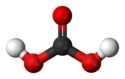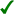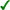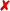# Carbonic acid

﻿
Carbonic acid
Not to be confused with carbolic acid, an antiquated name for phenol.
Carbonic acid is also an archaic name for carbon dioxide
Carbonic acidIdentifiers
CAS number 463-79-6ChemSpider 747KEGG C01353ChEBI CHEBI:28976ChEMBL CHEMBL1161632Jmol-3D images Image 1
Properties
Molecular formula H2CO3
Molar mass 62.03 g/mol
Density 1.0 g/cm3 (dilute soln.)
Melting point

n/a

Solubility in water Exists only in solution
Acidity (pKa) 6.352 (pKa1)acid (verify) (what is:/?)
Except where noted otherwise, data are given for materials in their standard state (at 25 °C, 100 kPa)
Infobox references

Carbonic acid is the inorganic compound with the formula H2CO3 (equivalently OC(OH)2). It is also a name sometimes given to solutions of carbon dioxide in water, because such solutions contain small amounts of H2CO3, and reactions proceed as if the main species was H2CO3. Carbonic acid forms two kinds of salts, the carbonates and the bicarbonates. It is a weak acid.

## Chemical equilibrium

When carbon dioxide dissolves in water it exists in chemical equilibrium producing carbonic acid:

CO2 + H2OH2CO3

The hydration equilibrium constant at 25 °C is called Kh, which in the case of carbonic acid is [H2CO3]/[CO2] = 1.70×10−3: hence, the majority of the carbon dioxide is not converted into carbonic acid, remaining as CO2 molecules. In the absence of a catalyst, the equilibrium is reached quite slowly. The rate constants are smaller for the forward reaction (CO2 + H2O → H2CO3) than for the reverse reaction (H2CO3 → CO2 + H2O). Carbonic acid is used in the making of soft drinks, inexpensive and artificially carbonated sparkling wines, and other bubbly drinks. The addition of two equivalents of water to CO2 would give orthocarbonic acid, C(OH)4, which exists only in minute amounts in aqueous solution.

Addition of base to an excess of carbonic acid gives bicarbonate. With excess base, carbonic acid reacts to give carbonate salts.

## Role of carbonic acid in blood

Carbonic acid is an intermediate step in the transport of CO2 out of the body via respiratory gas exchange. The hydration reaction of CO2 is generally very slow in the absence of a catalyst, but red blood cells contain carbonic anhydrase, which both increases the reaction rate and dissociates a hydrogen ion (H+) from the resulting carbonic acid, leaving bicarbonate (HCO3-) dissolved in the blood plasma. This catalysed reaction is reversed in the lungs, where it converts the bicarbonate back into CO2 and allows it to be expelled. This equilibration plays an important role as a buffer in mammalian blood.

## Role of carbonic acid in ocean chemistry

The oceans of the world have absorbed approximately one-third of the CO2 emitted by humans from the burning of fossil fuels.  The extra dissolved carbon dioxide has caused the ocean's average surface pH to shift by about 0.1 unit from pre-industrial levels. This process is known as ocean acidification.

## Acidity of carbonic acid

Carbonic acid is diprotic: it has two protons, which may dissociate from the parent molecule. Thus there are two dissociation constants, the first one for the dissociation into the bicarbonate (also called hydrogen carbonate) ion HCO3:

H2CO3HCO3 + H+
Ka1 = 4.45×10−7 ; pKa1 = 6.352 at 25 °C.

With a pKa1 of 6.352, carbonic acid H2CO3 is almost 10x weaker acid than acetic acid.

The second for the dissociation of the bicarbonate ion into the carbonate ion CO32−:

HCO3CO32− + H+
Ka2 = 4.69×10−11 ; pKa2 = 10.329 at 25 °C and Ionic Strength = 0.0.

Care must be taken when quoting and using the first dissociation constant of carbonic acid. In aqueous solution carbonic acid only exists in equilibrium with carbon dioxide, and the concentration of H2CO3 is much lower than the dissolved CO2 concentration. Since it is not possible to distinguish between H2CO3 and dissolved CO2 (referred to as CO2(aq)) by conventional methods, H2CO3* is used to represent the two species when writing the aqueous chemical equilibrium equation. The equation may be rewritten as follows (cf. sulfurous acid):

H2CO3*HCO3 + H+
Ka = 4.6×10−7(General Chemistry: An Integrated Approach Third Edition); pKa = 6.352 at 25 °C and Ionic Strength = 0.0.(NIST CRITICAL Database)

Whereas this pKa is quoted as the dissociation constant of carbonic acid, it is ambiguous: it might better be referred to as the acidity constant of dissolved carbon dioxide, as it is particularly useful for calculating the pH of CO2-containing solutions.

### pH and composition of carbonic acid solutions

At a given temperature, the composition of a pure carbonic acid solution (or of a pure CO2 solution) is completely determined by the partial pressure$p_{CO_2}$ of carbon dioxide above the solution. To calculate this composition, account must be taken of the above equilibria between the three different carbonate forms (H2CO3, HCO3 and CO32−) as well as of the hydration equilibrium between dissolved CO2 and H2CO3 with constant$K_h=\frac{[H_2CO_3]}{[CO_2]}$ (see above) and of the following equilibrium between the dissolved CO2 and the gaseous CO2 above the solution:

CO2(gas)CO2(dissolved) with$\frac{[CO_2]}{p_{CO_2}}=\frac{1}{k_\mathrm{H}}$ where kH=29.76 atm/(mol/L) at 25°C (Henry constant)

The corresponding equilibrium equations together with the$\scriptstyle[H^+][OH^-]=10^{-14}$ relation and the charge neutrality condition$\scriptstyle[H^+]=[OH^-]+[HCO_3^-]+2[CO_3^{2-}]$ result in six equations for the six unknowns [CO2], [H2CO3], [H+], [OH], [HCO3] and [CO32−], showing that the composition of the solution is fully determined by$p_{CO_2}$. The equation obtained for [H+] is a cubic whose numerical solution yields the following values for the pH and the different species concentrations:$p_{CO_2}$
(atm)
pH [CO2]
(mol/L)
[H2CO3]
(mol/L)
[HCO3]
(mol/L)
[CO32−]
(mol/L)
1.0 × 10−8 7.00 3.36 × 10−10 5.71 × 10−13 1.42 × 1009 7.90 × 10−13
1.0 × 10−7 6.94 3.36 × 1009 5.71 × 10−12 5.90 × 1009 1.90 × 10−12
1.0 × 10−6 6.81 3.36 × 1008 5.71 × 10−11 9.16 × 1008 3.30 × 10−11
1.0 × 10−5 6.42 3.36 × 1007 5.71 × 1009 3.78 × 1007 4.53 × 10−11
1.0 × 10−4 5.92 3.36 × 1006 5.71 × 1009 1.19 × 1006 5.57 × 10−11
3.5 × 10−4 5.65 1.18 × 1005 2.00 × 1008 2.23 × 1006 5.60 × 10−11
1.0 × 10−3 5.42 3.36 × 1005 5.71 × 1008 3.78 × 1006 5.61 × 10−11
1.0 × 10−2 4.92 3.36 × 1004 5.71 × 1007 1.19 × 1005 5.61 × 10−11
1.0 × 10−1 4.42 3.36 × 1003 5.71 × 1006 3.78 × 1005 5.61 × 10−11
1.0 × 10+0 3.92 3.36 × 1002 5.71 × 1005 1.20 × 1004 5.61 × 10−11
2.5 × 10+0 3.72 8.40 × 1002 1.43 × 1004 1.89 × 1004 5.61 × 10−11
1.0 × 10+1 3.42 3.36 × 1001 5.71 × 1004 3.78 × 1004 5.61 × 10−11
• We see that in the total range of pressure, the pH is always largely lower than pKa2 so that the CO32− concentration is always negligible with respect to HCO3 concentration. In fact CO32− plays no quantitative role in the present calculation (see remark below).
• For vanishing$p_{CO_2}$, the pH is close to the one of pure water (pH = 7) and the dissolved carbon is essentially in the HCO3 form.
• For normal atmospheric conditions ($p_{CO_2}=3.5\times 10^{-4}$ atm), we get a slightly acid solution (pH = 5.7) and the dissolved carbon is now essentially in the CO2 form. From this pressure on, [OH] becomes also negligible so that the ionized part of the solution is now an equimolar mixture of H+ and HCO3.
• For a CO2 pressure typical of the one in soda drink bottles ($p_{CO_2}$ ~ 2.5 atm), we get a relatively acid medium (pH = 3.7) with a high concentration of dissolved CO2. These features contribute to the sour and sparkling taste of these drinks.
• Between 2.5 and 10 atm, the pH crosses the pKa1 value (3.60) giving a dominant H2CO3 concentration (with respect to HCO3) at high pressures.

Remark

As noted above, [CO32−] may be neglected for this specific problem, resulting in the following very precise analytical expression for [H+]:$\scriptstyle[H^+] \simeq \left( 10^{-14}+\frac {K_hK_{a1}}{k_\mathrm{H}} p_{CO_2}\right)^{1/2}$

## Spectroscopic studies of carbonic acid

Theoretical calculations show that the presence of even a single molecule of water causes carbonic acid to revert to carbon dioxide and water. In the absence of water, the dissociation of gaseous carbonic acid is predicted to be very slow, with a half-life of 180,000 years.

It has long been recognized that pure carbonic acid cannot be obtained at room temperatures (about 20 °C or about 70 °F). It can be generated by exposing a frozen mixture of water and carbon dioxide to high-energy radiation, and then warming to remove the excess water. The carbonic acid that remained was characterized by infrared spectroscopy. The fact that the carbonic acid was prepared by irradiating a solid H2O + CO2 mixture may suggest that H2CO3 might be found in outer space, where frozen ices of H2O and CO2 are common, as are cosmic rays and ultraviolet light, to help them react. The same carbonic acid polymorph (denoted beta-carbonic acid) was prepared by heating alternating layers of glassy aqueous solutions of bicarbonate and acid in vacuo, which causes protonation of bicarbonate, followed by removal of the solvent. Alpha-carbonic acid was prepared by the same technique using methanol rather than water as a solvent.

Wikimedia Foundation. 2010.

Synonyms:

### Look at other dictionaries:

• Carbonic acid — Carbonic Car*bon ic, a. [Cf. F. carbonique. See {Carbon}.] (Chem.) Of, pertaining to, or obtained from, carbon; as, carbonic oxide. [1913 Webster] {Carbonic acid} (Chem.), an acid {HO.CO.OH}, not existing separately, which, combined with positive …   The Collaborative International Dictionary of English

• carbonic acid — ► NOUN ▪ a very weak acid formed when carbon dioxide dissolves in water …   English terms dictionary

• carbonic acid — n. a weak, colorless acid, H2CO3, formed by the solution of carbon dioxide in water and existing only in solution …   English World dictionary

• carbonic acid — H2CO3, formed from H2O and CO2. * * * carbonic acid n a weak dibasic acid H2CO3 known only in solution that reacts with bases to form carbonates * * * car·bon·ic ac·id (kahr bonґik) the chemical species H2CO3, which exists in chemical equilibrium …   Medical dictionary

• carbonic acid — Choke damp, fixed air, foul air, mephitic air, carbonic acid gas …   New dictionary of synonyms

• carbonic acid — noun a weak acid known only in solution; formed when carbon dioxide combines with water • Derivationally related forms: ↑carbonate • Hypernyms: ↑acid …   Useful english dictionary

• carbonic acid — noun Date: 1788 a weak dibasic acid H2CO3 known only in solution that reacts with bases to form carbonates …   New Collegiate Dictionary

• carbonic acid — the acid, H2CO3, formed when carbon dioxide dissolves in water, known in the form of its salts and esters, the carbonates. [1785 95] * * * …   Universalium

• carbonic acid — noun a weak unstable acid, HCO, known only in solution, and as carbonate salts; it is present in carbonated drinks, and sparkling wine, but decomposes to form …   Wiktionary

• carbonic acid — weak unsteady and colorless acid that is existent only in solution and is produced by dissolving carbon dioxide in water …   English contemporary dictionary